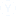ans plz ....
twowomen ganga andsaraswati ,working separately canmown a field in 8 and 12 hrsrespectively . if they work instretches of one hour alternatelynga beginning at 9am ,when willthe moving be finished.
• MC
• Mohini
• 21 अप्रैल
• 1183 दृश्य
• 4 उत्तर4 उत्तर
1-4 of  4
4 उत्तर
• yah 6.30pm

• 12*8/12+8= 4.8... 4.8*2= 9.6 hour... 9 Am + 9.6 hr= 18 hr (6*60/10)... 18hr 36 minutes = 6 Pm 36 minutes....

• Ganga work is 8 hrs, while saraswathi is 12hrs. Avg of 8 & 12 is 24. So let total work be 24units. Ganga can do 3 units/hr ; Saras can do 2units/hr. On combination it is 5units. Since they work alternatively. So it's 5units/2hours. Which give 20units in 10hours. (ie. 5pm). Remaining 4units , where 1st 3 units by Ganga which is 1 Hr. ( ie. now 6pm) and 1 unit by Sara which is 30mins. So Ans. 6pm30mins

• Whenever, workers are working alternatively on one work, we take 2 as 1 unit. In this case, we take 2 hours as 1 unit. Part of the field moved by Ganga and Saraswati in 2 hours (1 unit) = 1/8 + 1/12 = 5/24; Time taken to complete the work, = 1/(5/24) = 24/5 unit of time; Then actual time taken by them to complete the work, = 24*2/5 = 9.5 hours. The work starts at 9 a.m. then it will complete at 6:30 pm.अन्य संबंधित चर्चाएंमॉक परीक्षण अभ्यास के लिए
sbi po prelim exam